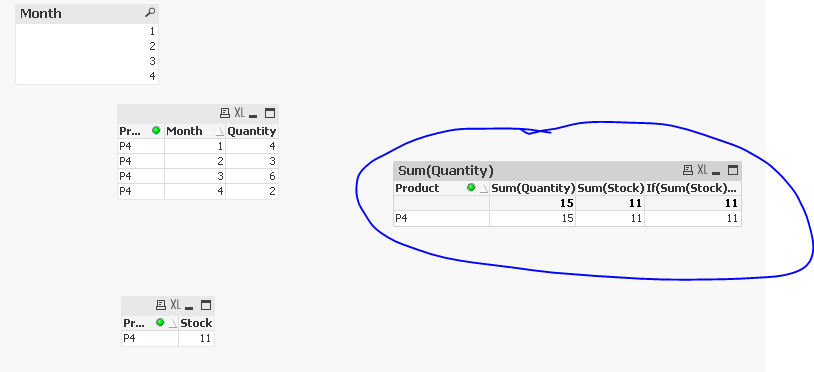QlikView App Dev

Discussion Board for collaboration related to QlikView App Development.

Announcements
QlikWorld 2022, LIVE in Denver CO., May 16-19, 2022. REGISTER NOW TO RECEIVE EARLY BIRD PRICING
cancel
Showing results for
Did you mean:Not applicable

Set input field with expression from chart

Hello !

I have an input field initialized with zero.

I want to set a value on it based on a expression of a chart, and only then the user can adjust it.

I need that because the inicial calculation of this field is based on a sum of selected items.

This what I have:

Inventory:

ProductStock
P110
P23
P39
P411

Sells History:

ProductMonthQuantity
P113
P124
P132
P243
P315
P324
P414
P423
P436
P442

Demand:

ProductSum(Quantity)Allocated
P177
P243
P399
P477

My inputfield is "Allocated". In this example, I selected the Months 1 and 2.

The initial expression for it is:

If(Sum(Stock) >= Sum(Quantity), Sum(Quantity), Sum(Stock))

For the Product P1, the "initial value" of the input field "Allocated" is 7 (because the Sum(Quantity) is based on Months 1 and 2).

If I change the selection of the Months, the initial value of the input field "Allocated" should change.

How can I achieve this ?

Thanks!!

Josué

4 RepliesMVP

I don't really understand the requirement clearly, would you be able to share the output you are expecting based on few different selections?Not applicable
Author

Let's consider that the value of Sum(Stock) of Product P1 is 8 (Ignore the first table).

If I select the Months 1 and 2, the Sum(Quantity) of product P1 is 7 (see second table).

So, following the expression for the initial value of "Allocated" of Product P1, its value should be 7.

If I select Months 1, 2 an 3, the Sum(Quantity) of Product P1 is 9 (3 + 4 + 2).

With that, the initial value of "Allocated" should be 8 (according to the formula expression).MVP

I think your formula is doing that. Look at P4 Product

Picking stock, since Sum(Stock) is lower when no selection is made.

Picking Quanitity, since Sum(Stock) is higher now based on Month 1 and 2 selectedIsn't that what you want?Not applicable
Author

Yes, but I need that inside an input field.

My idea (actually the client's idea) is the following:

The user select a couple of months, then we will have an "initial" value for "Allocated" (based on the expression).

Then, he can adjust this value manually.

As the expression is based on selections, I cannot set an initial value for this field using the load scripts.Community Browser